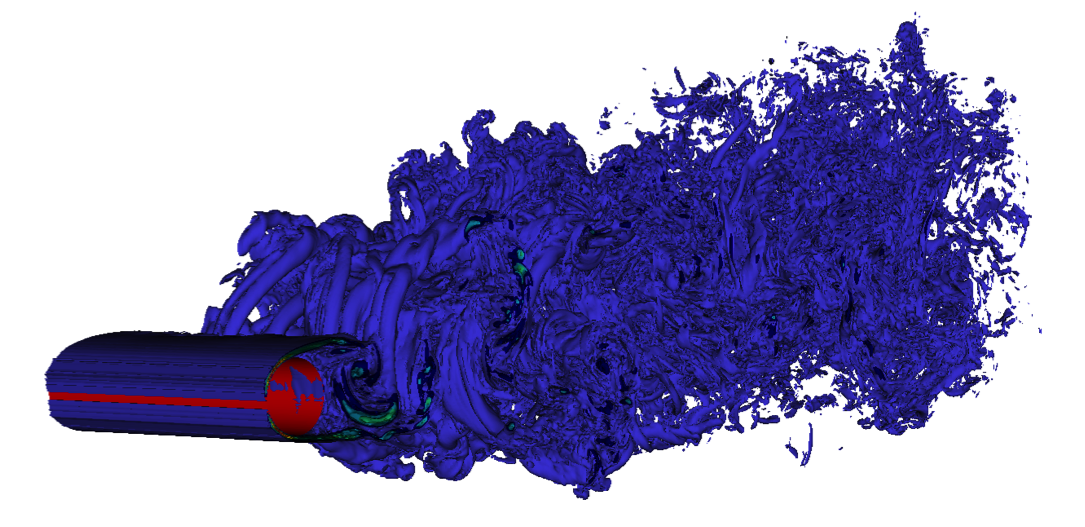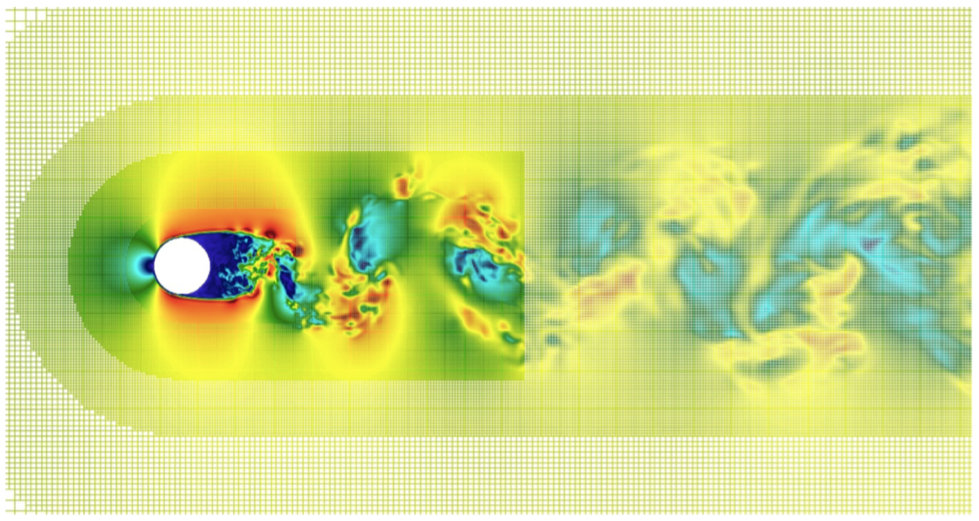EN FR
EN FR
##### MEMPHIS - 2018
Research Program
Application Domains
New Software and Platforms
Partnerships and Cooperations
Bibliography
Research Program
Application Domains
New Software and Platforms
Partnerships and Cooperations
Bibliography

## Section: Highlights of the Year

### Highlights of the Year

#### Turbulent flow simulations using Octrees

We have initially developed and tested a 3D first-order Octree code for unsteady incompressible Navier-Stokes equations for full windmill simulations with an LES model and wall laws. We have validated this code on Occigen for complex flows at increasing Reynolds numbers. This step implied identifying stable and feasible schemes compatible with the parallel linear Octree structure. The validation has been conducted with respect to the results of a fully Cartesian code (NaSCAR) that we run on Turing (with significantly more degrees of freedom) and with respect to experimental results.

Subsequently, we have developed a second-order Octree scheme that has been validated on Occigen for a sphere at a moderate Reynolds number ($\mathrm{Re}=500$), see Table 1. Then, for a cylinder at ($\mathrm{Re}=140000$) (Figures 5(a) and 5(b)), close to real applications, we have preliminary validation results for the second-order scheme with respect to experimental drag coefficient (Table 2). Additional resources will be asked on Occigen to complete the study.

 Mesh $\Delta {x}_{\mathrm{min}}$ number of cells ${C}_{\mathrm{D}}$ (1st-order scheme) ${C}_{\mathrm{D}}$ (2nd-order scheme) 1 $0.094$ $0.72·{10}^{5}$ N.A. $0.526$ 2 $0.047$ $4.9·{10}^{5}$ $0.595$ $0.522$ 3 $0.023$ $4.7·{10}^{6}$ $0.546$ $0.492$ 4 $0.012$ $37.6·{10}^{6}$ $0.555$ $0.496$
 Case ${C}_{\mathrm{D}}$ Octree, 1st-order scheme $1.007$ Octree, 2nd-order scheme $1.157$ Cartesian $1.188$ Experimental estimate  $1.237$
Figure 5. flow past a cylinder at $\mathrm{Re}=140000$. Left: vorticity contour lines. Right: streamwise velocity section and grid for the second-order Octree scheme.